# Perform Math Calculations in Flash

By Scott Manning
Web
Share:

The days of Flash being used solely for entertainment are over. Macromedia has spent an enormous amount of money and effort to bring Flash out of its “animation only” box, and promote it as a cross-platform development environment, as well as a designer environment. Along with the funny cartoons, Flash can now be used to build business applications.

One of the basic requirements of any business app is the ability to do math. In this tutorial, we will cover how to do basic arithmetic in Flash. We’ll also cover the capture of data input by a user, and learn how to perform calculations based on this data.

##### The Search for Information on Flash’s Calculation Abilities

A little over a year ago, I started a project that required Flash to do some serious calculations. I knew that calculations were possible in Flash; it was just that I’d never done any. So, like any good Web user, I started to search forums and Google for some guidance. Unfortunately, I found very little information on what I was looking for.

I then went to my local bookstore in search of answers, but found the same problem: there is virtually no documentation available on using math in real world Flash applications.

Don’t believe me? Search on Macromedia.com for the term “calculation”. Go to your local bookstore, look at any book claiming to be the be-all and end-all and for Flash, and see what you can find under “math” or “equations”. Sure, there are plenty of examples the explain the use of math to make random swirling lines, flying squares, and dancing midgets, but what about using math to calculate data? Those dancing midgets are cute, but calculating data can make money.

After looking through about 30 books, I found two that offered about a page on what I was looking for. It wasn’t much, but it was something.

If Rich Internet Applications are going to be as big as Macromedia hopes, more tutorials and examples covering basic, real-world business needs like calculating data will need to be written. This tutorial is the first attempt to pique developer awareness of, and interest in, Flash’s math capabilities.

Flash can handle any equation you throw at it, from simple addition, to complex calculus.

##### Static Calculations

To get you acquainted with what Flash can do, we’ll start off with simple, static calculations. By “static”, I mean equations that are hard coded, and do not require any input from the end user.

All these calculations are performed with ActionScript, so we’ll be doing a little coding. Let’s go!

1. Start up Flash, and create a new movie.
2. Draw four dynamic textboxes on your stage. The dynamic property is set on the Properties panel.3. Once that’s done, give each textbox a Variable name. In this example, I’m using “addition”, “subtraction”, “multiplication”, and “division” as the Variable names.Tip: When developing, you should always give objects (textboxes, components, Movieclips, etc.) unique names. Otherwise, you run the risk of confusing Flash. This would be like having two brothers named John and John, and having you mom say, "Tell John to wash the car and tell John to clean the bathroom.” Avoid the confusion by giving every object a unique name.

We’ll be using these variables to tell Flash were to display the results of our calculations.

4. In the timeline, create a new layer. Name the layer containing the textboxes “Calculations”, and name the new layer “Actions”. We do this so that we can easily see which layer contains the code, and which layer contains the user interface.5. Now, let’s apply the calculations. Select the first frame in your Actions layer. In order to give ourselves freedom to type what we wish, we need to set the Actions panel to Expert Mode. Choose Expert Mode from the Actions panel pop-up menu (at the upper right of the panel).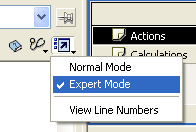6. Now, input the following code:
``````addition = 1+1;
subtraction = 5-2;
multiplication = 10*2;
division = 100/5;``````

Now, an explanation of what this code does and why. There are four lines, and at the beginning of each is a Variable reference. Remember the Variable names we gave to the four textboxes? The first line starts with “addition”. This references our “addition” textbox. We then give an expression to which "addition” is equal. The “addition” textbox will display the results of one plus one, the subtraction textbox will display the results of five minus two, etc.

Publish your movie to see the results!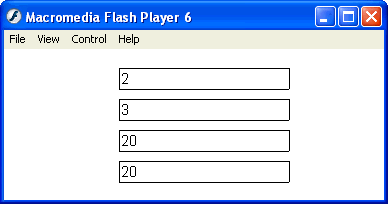If you get really lost, here’s a sample FLA that has all the details.

Now that you understand how to perform basic calculations, mixing them up is just a matter of algebra. The same math rules apply.

``sample = 10*2-6/3;``

The above sample would give you a result of 18.

As in algebra, multiplications and divisions are calculated before additions and subtractions, and the use of parentheses specifies that anything in the parentheses will be calculated first. Using parentheses can deliver different results. For example, 10*(3-2) will give you a result of 10, where 10*3-2 will give you a result of 28.

The ActionScript in Flash would look like this:

``sample = 10(3-2);``

Calculating User-Entered Data

Calculating static data is helpful, but calculating data that’s input by a user is powerful and sellable. The possibilities are endless, but here, we’ll cover basic data entry and calculation. Our sample will take two numbers entered by the user. On the click of a button, the Flash movie will display the sum, and the product of the numbers.

1. Create a new movie.
2. Create four textboxes in the first frame of the movie, and arrange them vertically.
3. In the Properties panel, set the textboxes to be left-aligned. The top two textboxes should be Input text, while the bottom two should be Dynamic text.
4. Limit the textboxes to numbers only. To do this, just select a textbox and click the Character… button in the Properties panel. This will bring up the Character Options box. From there, select “Only”, “Numerals (0-9)”, and click Done.5. I’ve added a line in the middle of my textboxes, to give them some sex appeal!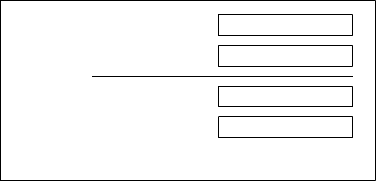6. Next, add some static text to the left of the text boxes. Starting from the top to the bottom:
• Number 1
• Number 2
• Sum of Number 1 and Number 2
• Product of Number 1 and Number 2

This is done to clearly label everything for the end user — us!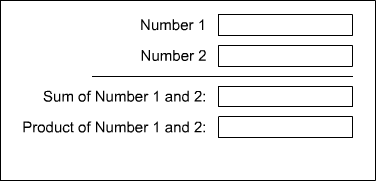7. The textboxes need to be given Variable names, so that Flash knows what we’re talking about when we refer to them. The Variable for a textbox is defined in the Properties panel. Starting from the top to the bottom, name the textboxes accordingly:
• number_one
• number_two
• result_sum
• result_product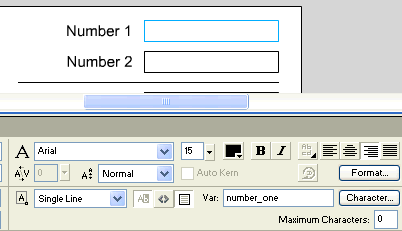8. Now, we need to add our button used to execute our calculations. In the Component panel, select Flash UI Components.
9. Drag and drop the PushButton component into the scene.10. Select the PushButton component you’ve just dragged into your scene. In the Properties panel, label the component “Calculate” and set the Click Handler to “onCalculate”. This is the button we will use to calculate the user’s data.11. As a final measure, I have given the “Number 1” and “Number 2” textboxes a value of zero. When you’re done, you’re scene should look something like the image below.Ok. We have our scene set up, so now, it’s time to put in the code that will make this movie work.

The Code

1. Name the current layer in the movie “content”. Add a new layer to the movie, and call it “actions”.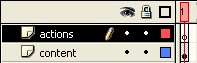2. Select the first frame of the “actions” layer. In the Actions panel, add the following code:
``````function onCalculate() {
one = Number(number_one);
two = Number(number_two);
result_sum = one + two;
result_product = one * two;
}``````

This is the code that will make this movie do its magic! Let’s look at what this code does and why.

``function onCalculate() {``

This first line starts a new function in the movie. Remember that we gave our PushButton component a Click Handler of “onCalculate”. When this button is clicked, it will execute the code within this function.

``````one = Number(number_one);
two = Number(number_two);``````

This code has two purposes. The first is to give shorter variable names to the data that’s being calculated. Instead of spelling out “number_one” throughout our code, we can now just use “one”.

This makes more sense when taken in conjunction with the second purpose: to treat these variables as numbers. This is done with Number(), which tells Flash that we to treat values in parentheses as numbers. If we don’t, when we calculate 1 plus 1, we’ll get 11. Or, if we calculate 1 plus 2, we’ll get 12. Instead, with the Number(), when we calculate 1 plus 1, we’ll get 2.

``````result_sum = one + two;
result_product = one * two;
}``````

Remember the variable names we gave the two textboxes at the bottom? This code puts our calculation results within those textboxes. When this code is executed, the “result_sum” textbox will display the result of adding Number 1 and Number 2. The “result_product” textbox will display the result of multiplying them.

That’s it! Publish your movie, type in some numbers in first two textboxes, and hit Calculate.